### Constrained Optimization

A function that plays a pivotal role in establishing conditions that characterize a local minimum of an NLP problem is the Lagrangian function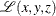, which is defined as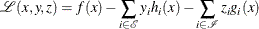Note that the Lagrangian function can be seen as a linear combination of the objective and constraint functions. The coefficients of the constraints,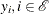, and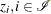, are called the Lagrange multipliers or dual variables. At a feasible point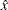, an inequality constraint is called active if it is satisfied as an equality—that is,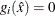. The set of active constraints at a feasible pointis then defined as the union of the index set of the equality constraints,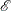, and the indices of those inequality constraints that are active at; that is,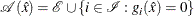An important condition that is assumed to hold in the majority of optimization algorithms is the so-called linear independence constraint qualification (LICQ). The LICQ states that at any feasible point, the gradients of all the active constraints are linearly independent. The main purpose of the LICQ is to ensure that the set of constraints is well-defined in a way that there are no redundant constraints or in a way that there are no constraints defined such that their gradients are always equal to zero.

#### The First-Order Necessary Optimality Conditions

If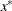is a local minimum of the NLP problem and the LICQ holds at, then there are vectors of Lagrange multipliers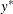and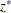, with components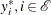, and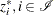, respectively, such that the following conditions are satisfied: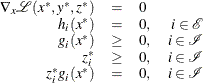where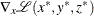is the gradient of the Lagrangian function with respect to x, defined as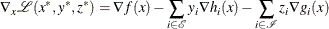The preceding conditions are often called the Karush-Kuhn-Tucker (KKT) conditions. The last group of equations (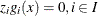) is called the complementarity condition. Its main aim is to try to force the Lagrange multipliers,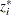, of the inactive inequalities (that is, those inequalities with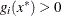) to zero.

The KKT conditions describe the way the first derivatives of the objective and constraints are related at a local minimum. However, they are not enough to fully characterize a local minimum. The second-order optimality conditions attempt to fulfill this aim by examining the curvature of the Hessian matrix of the Lagrangian function at a point that satisfies the KKT conditions.

#### The Second-Order Necessary Optimality Condition

Letbe a local minimum of the NLP problem, and letandbe the corresponding Lagrange multipliers that satisfy the first-order optimality conditions. Then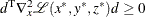for all nonzero vectors d that satisfy the following conditions:

1.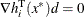,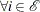2.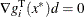,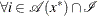, such that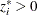3.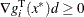,, such that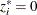The second-order necessary optimality condition states that, at a local minimum, the curvature of the Lagrangian function along the directions that satisfy the preceding conditions must be nonnegative.